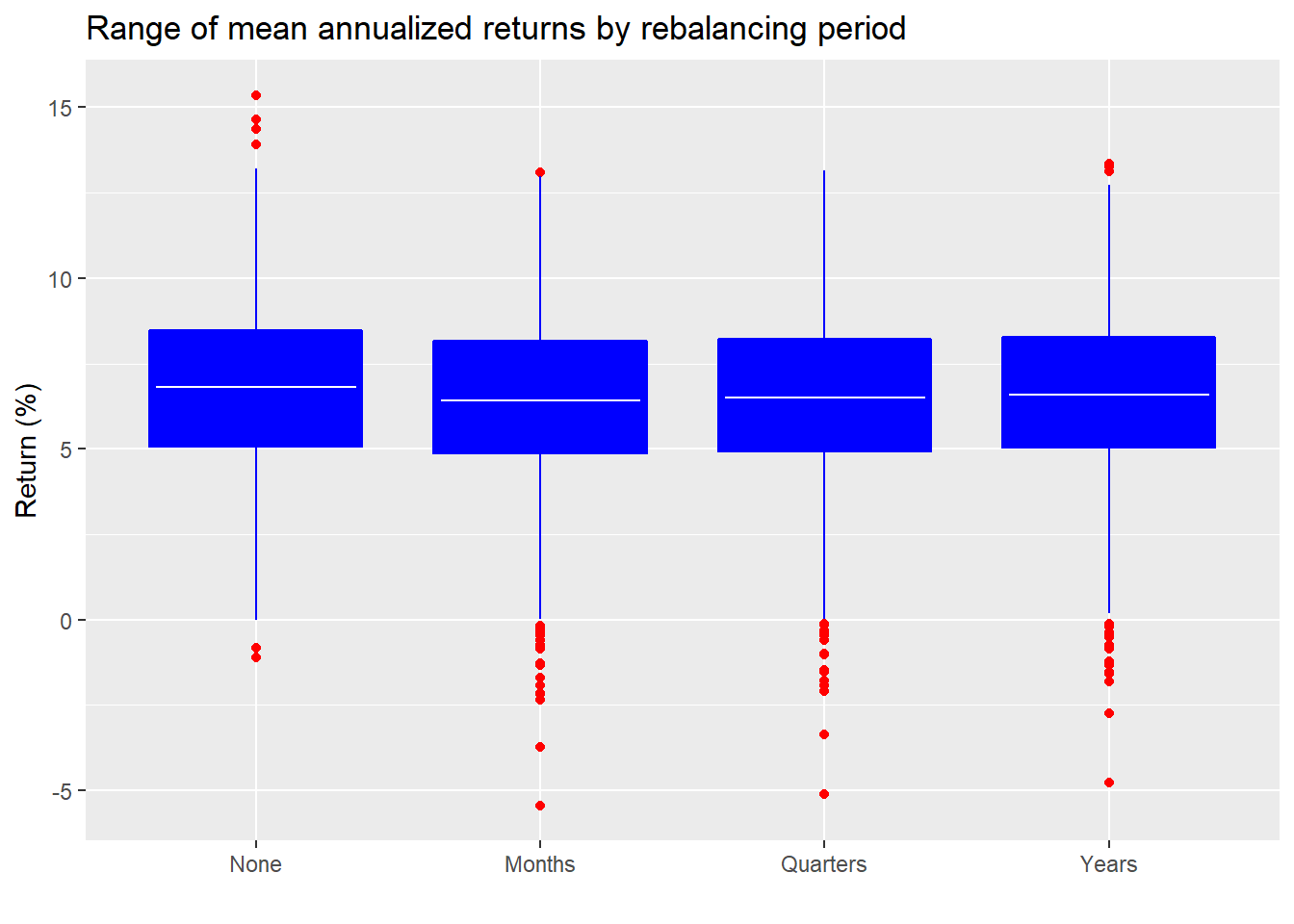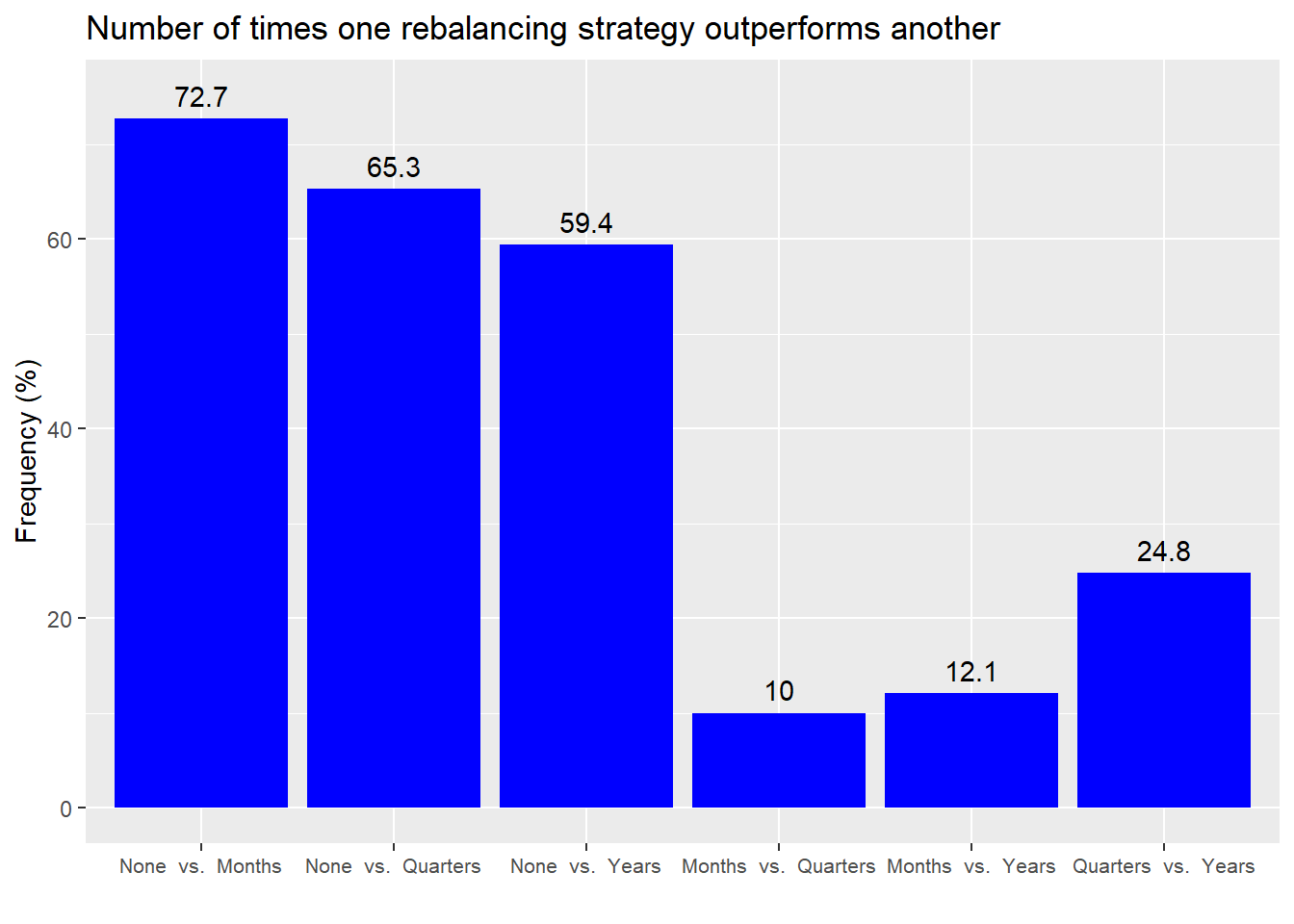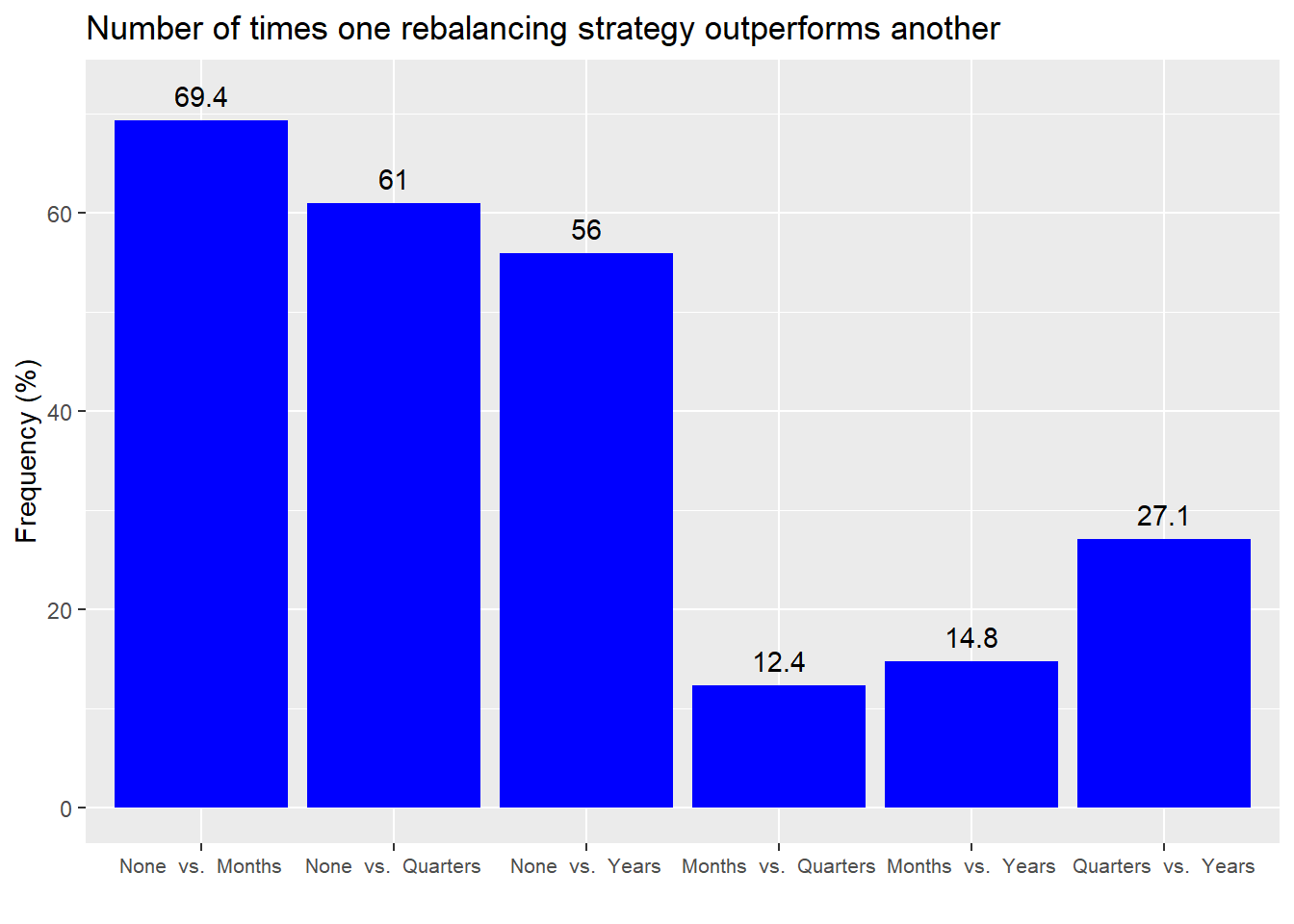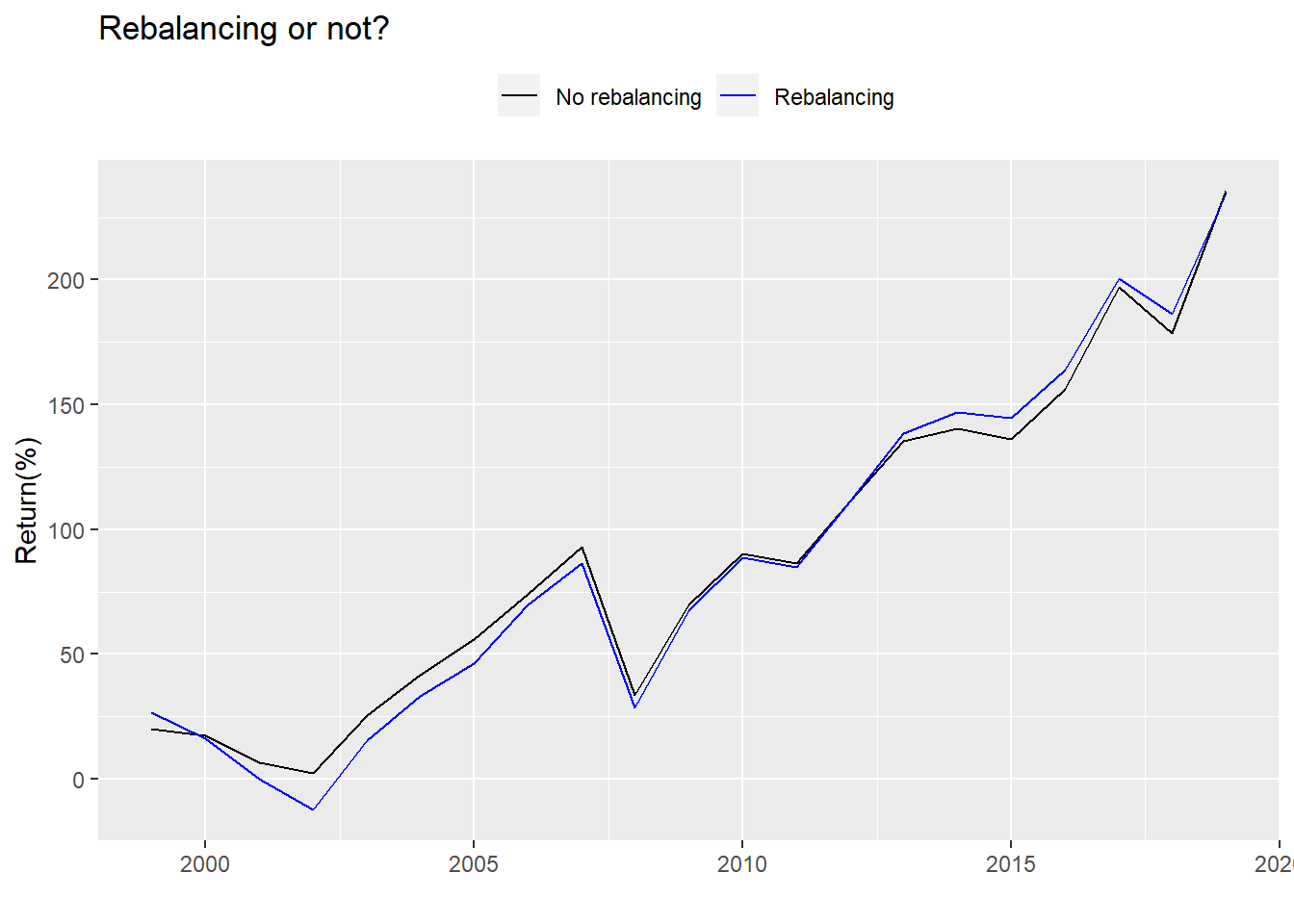# Rebalancing history

Our last post on rebalancing struck an equivocal note. We ran a thousand simulations using historical averages across different rebalancing regimes to test whether rebalancing produced better absolute or risk-adjusted returns. The results suggested it did not. But we noted many problems with the tests—namely, unrealistic return distributions and correlation scenarios. We argued that if we used actual historical data and sampled from it, we might resolve many of these issues. But we also asked our readers whether it was worthwhile to test further. Based on the responses and page views, we believe the interest is there, so we’ll proceed!

As we mentioned, historical data more closely approximates the fat-tailed, skewed distribution common to asset returns. But only if you have a long enough time series. While we weren’t able to find 50 years worth of major asset class returns, we were able to compile a 20-year series that includes two market downturns. The data isn’t all from the same source, unfortunately. But it is fairly reputable—Vanguard’s stock and US bond index funds, emerging market bond indices from the St. Louis Fed, and the S&P GSCI commodity index. The code will show how we aggregated it for those interested. Using this data series we should be able to test rebalancing more robustly.

Before we proceed, a brief word on methodology. To run the simulation, we need to sample (with replacement) from our twenty year period and combine each sample into an entire series. To capture the non-normal distribution and serial correlation of asset returns, we can’t just sample one return, however. We need to sample a block of returns. This allows us to approximate the serial correlation of individual assets as well as the correlation between assets. But how long should the block be? Trying to answer that can get pretty complicated, pretty quickly.1 We decided to take a shortcut and use a simple block of 6 periods. This equates to six months, since our series is monthly returns. There’s nothing magical about this number but it does feature as a period used in academic studies on momentum, a topic beyond the scope of this post.2

We sample six months of returns at at time. Repeat 42 times to get over 20 years of data. Repeat to create 1000 portfolios. From there we apply the different rebalancing regimes on each of the portfolios and then aggregate the data. As before, we first use an equal-weighting, and then a 60/35/5 weighting for stocks, bonds, and commodities. Let’s see what we get.

First, we look at the average return for equal-weighted portfolios by rebalancing regime along with the range of outcomes.Recall, the white line is the mean and the top and bottom of the boxes represent the middle 50% of outcomes, Interestingly, no rebalancing had far more positive and far less negative outliers (the red dots) than any of the rebalancing regimes.

Given where the averages line up, it doesn’t look like there are significant differences. Let’s run some t-tests for completeness.

Table 1: Aggregate p-values for simulation
Comparison P-value
None vs. Months 0.87
None vs. Quarters 0.87
None vs. Years 0.88
Months vs. Quarters 0.97
Months vs. Years 0.95
Quarters vs. Years 0.97

As expected, the p-values are quite high, meaning that any differences in mean returns are likely due to chance.

Now we’ll check on the number of times each rebalancing strategy outperforms the others.A dramatic result! No rebalancing beat the other strategies a majority of the time and less rebalancing outperformed more most of the time too. Now for the crux. Does rebalancing lead to better risk-adjusted returns as calculated by the Sharpe ratio.

Table 2: Sharpe ratios by rebalancing period
Period Ratio
None 0.76
Months 0.74
Quarters 0.75
Years 0.76

Not much difference. Recall that from our previous simulations, no rebalancing actually generated a slightly worse Sharpe ratio by about 30-40 bps. But that result occurred less than 90% of the time, so it could be due to randomness. Let’s check the Sharpe ratios for the present simulation.

Table 3: Frequency of a better Sharpe ratio (%)
Periods Occurence
None vs. Months 60.2
None vs. Quarters 53.4
None vs. Years 48.3
Months vs. Quarters 3.7
Months vs. Years 8.2
Quarters vs. Years 22.1

No rebalancing generates a better Sharpe ratio a majority of the time, but not enough to conclude it isn’t due to chance. Interestingly, the frequency with which quarterly and yearly rebalancing produce better Sharpe ratios than monthly rebalancing looks significant. In both cases the frequency is greater than 90% of the time. That the lower frequency rebalancing outperforms the higher frequency likely plays a role in the significance of the Sharpe ratios, but is an area of investigation we’ll shelve for now.

Let’s move to the next simulation where we weight the portfolios 60/35/5 for stocks, bonds, and commodities. First, we show the boxplot of mean returns and range of outcomes.Like the equal-weighted simulations, the means don’t look that dissimilar and no rebalancing generates more positive and less negative outliers than other rebalancing regimes. We can say almost undoubtedly that the differences in average returns, if there are any, is likely due to chance. The p-values from the t-tests we show below should prove that.

Table 4: Aggregate p-values for simulation
Comparison P-value
None vs. Months 0.91
None vs. Quarters 0.92
None vs. Years 0.92
Months vs. Quarters 0.98
Months vs. Years 0.97
Quarters vs. Years 0.98

Now let’s calculate and present the frequency of outperformance by rebalancing strategy.No rebalancing outperforms again! Less frequent rebalancing outperforms more frequent. And risk-adjusted returns?

Table 5: Sharpe ratios by rebalancing period
Period Ratio
None 0.66
Months 0.66
Quarters 0.67
Years 0.68

Here, no rebalancing performed slightly worse than rebalancing quarterly or yearly. How likely should we believe these results to be significant?

Table 6: Frequency of a better Sharpe ratio (%)
Periods Occurence
None vs. Months 47.8
None vs. Quarters 40.9
None vs. Years 35.5
Months vs. Quarters 2.2
Months vs. Years 6.4
Quarters vs. Years 21.3

Slightly worse than 50/50 for no rebalancing. But less frequent rebalancing appears to have the potential to produce higher risk-adjusted returns that more frequent rebalancing.

Let’s briefly sum up what we’ve discovered thus far. Rebalancing does not seem to produce better risk-adjusted returns. If we threw in taxation and slippage, we think rebalancing might likely be a significant underperformer most of the time.

Does this mean you should never rebalance? No. You should definitely rebalance if you’ve got a crystal ball. Baring that, if your risk-return parameters change, then you should rebalance. But it would not be bringing the weights back to their original targets; rather, it would be new targets. A entirely separate case.

What do we see as the biggest criticism of the foregoing analysis? That it was a straw man argument. In practice, few professional investors rebalance because it’s July 29th or October 1st. Of course, there is quarter-end and year-end rebalancing, but those dates are usually coupled with a threshold. That is, only rebalance if the weights have exceeded some threshold, say five or ten percentage points from target. Analyzing the effects of only rebalancing based on thresholds would require more involved code on our part.3 Given the results thus far, we’re not convinced that rebalancing based on the thresholds would produce meaningfully better risk-adjusted returns.

However, rebalancing based on changes in risk-return constraints might do so. Modeling that would be difficult since we’d also have to model (or assume) new risk-return forecasts. But we could model the traditional shift recommended by financial advisors to clients as they age; that is, slowly shifting from high-risk to low-risk assets. In other words, lower the exposure to stocks and increase the exposure to bonds.

As a toy example, we use our data set to compare a no rebalancing strategy with an initial 60/35/5 split between stocks, bonds, and commodities to a yearly rebalancing strategy that starts at a 90/5/5 split and changes to a 40/60/0 split over the period. The Sharpe ratio for the rebalanced portfolio is actually a bit worse than the no rebalancing one. Mean returns are very close. Here’s the graph of the cumulative return.This is clearly one example and highly time-dependent. But we see that rebalancing wasn’t altogether different than not, and we’re not including tax and slippage effects. To test this notion we’d have to run some more simulations, but that will be for another post.

We’ll end this post with a question for our readers. Are you convinced rebalancing doesn’t improve returns or do you think more analysis is required? Please send us your answer to nbw dot osm at gmail dot com. Until next time, here’s all the code behind the simulations, analyses, and charts.

## Load packages
library(tidyquant)
library(tidyverse)

## Stocks
symbols <- c("VTSMX", "VGTSX", "VBMFX", "VTIBX")
prices <- getSymbols(symbols, src = "yahoo",
from = "1990-01-01",
auto.assign = TRUE) %>%
reduce(merge) %>%
colnames<-(tolower(symbols))

## Bonds
# Source for bond indices:
# https://fred.stlouisfed.org/categories/32413

em_hg <- getSymbols("BAMLEMIBHGCRPITRIV",
src = "FRED",
from = "1990-01-01",
auto.assign = FALSE)

em_hg <- em_hg %>% na.locf()

em_hy <- getSymbols("BAMLEMHBHYCRPITRIV",
src = "FRED",
from = "1990-01-01",
auto.assign = FALSE)

em_hy <- em_hy %>% na.locf()

# Commodity data
# Source for commodity data
# https://www.investing.com/indices/sp-gsci-commodity-total-return-historical-data
# Unfortunately, the data doesn't open into a separate link so you'll need to download it into a
# csv file unless you're good a web scraping. We're not. Note too, the dates get a little funky
# when being transferred into the csv, so you'll need to clean that up. Finally, the dates are
# give as beginning of the month. But when we spot checked a few, they were actually end of the
# month, which lines up with the other data

cmdty$Date <- as.Date(cmdty$Date,"%m/%d/%Y")
cmd_price <- cmdty %>%
filter(Date >="1998-12-01", Date <="2019-12-31")

## Merged
merged <- merge(prices[,1:3], em_hg, em_hy)
colnames(merged) <- c("us_stock", "intl_stock", "us_bond", "em_hg", "em_hy")
merged <- merged["1998-12-31/2019-12-31"] %>% na.locf()

merge_mon <- to.monthly(merged, indexAt = "lastof", OHLC = FALSE)
merge_mon$cmdty <- cmd_price$Price
merge_yr <- to.yearly(merge_mon, indexAt = "lastof", OHLC = FALSE)

merge_ret <- ROC(merge_mon, type = "discrete") %>% na.omit()
merge_ret_yr <- ROC(merge_yr, type = "discrete") %>% na.omit()

## Data frame
df <- data.frame(date = index(merge_ret), coredata(merge_ret))
df_yr <- data.frame(date = index(merge_ret_yr), coredata(merge_ret_yr))

### Block sampling
## Create function
block_samp <- function(dframe,block,cycles){
idx <- seq(1,block*cycles,block)

assets <- ncol(dframe)
size <- block*cycles

mat <- matrix(rep(0,assets*size), ncol = assets)

for(i in 1:cycles){

start <-sample(size,1)

if(start <= (size - block + 1)){
end <- start + block -1
len <- start:end
}else if(start > (size - block + 1) & start < size){
end <- size
step <- block - (end - start) - 1
if(step == 1){
}else{
}

}else{
}

mat[idx[i]:(idx[i]+block-1),] <- data.matrix(df[len,2:7])
}

mat
}

# Create 1000 samples

set.seed(123)
block_list <- list()
for(i in 1:1000){
block_list[[i]] <- block_samp(df[,2:7], 6, 42)
}

### Rebalancing on simulation
## Create function

rebal_func <- function(port, wt, ...){

if(missing(wt)){
wt <- rep(1/ncol(port), ncol(port))
}else{
wt <- wt
}

port <- ts(port, start = c(1999,1), frequency = 12)

port_list <- list()
rebals = c("none","months", "quarters", "years")

for(pd in rebals){
if(pd == "none"){
port_list[[pd]] <- Return.portfolio(port, wt) %>%
colnames<-(pd)
}else{
port_list[[pd]] <- Return.portfolio(port, wt, rebalance_on = pd)%>%
colnames<-(pd)
}
}

port_r <- port_list %>%
bind_cols() %>%
data.frame()

port_r

}

## Run function on simulations
# Note this may take 10 minutes to run. We hope to figure out a way to speed this up in later
# versions.

rebal_test <- list()
for(i in 1:1000){
rebal_test[[i]] <- rebal_func(block_list[[i]])
}

### Analyze results
## Average results
rebal_mean_df <- data.frame(none = rep(0,1000),
monthly = rep(0,1000),
quarterly = rep(0,1000),
yearly = rep(0,1000))
for(i in 1:1000){
rebal_mean_df[i,] <- colMeans(rebal_test[[i]]) %>% as.vector()
}

port_names <-  c("None", "Months", "Quarters", "Years")

# Boxplot of reults
rebal_mean_df %>%
colnames<-(port_names) %>%
gather(key,value) %>%
mutate(key = factor(key, levels = port_names)) %>%
ggplot(aes(key,value*1200)) +
geom_boxplot(fill = "blue", color = "blue", outlier.colour = "red") +
stat_summary(geom = "crossbar", width=0.7, fatten=0, color="white",
fun.data = function(x){ return(c(y=mean(x), ymin=mean(x), ymax=mean(x))) })+
labs(x = "",
y = "Return (%)",
title = "Range of mean annualized returns by rebalancing period")

## Find percentage of time one rebalancing period generates higher returns than another
# Create means comparison function
freq_comp <- function(df){
count <- 1
opf <- data.frame(comp = rep(0,6), prob = rep(0,6))
port_names <-  c("None", "Months", "Quarters", "Years")

for(i in 1:4){
for(j in 2:4){
if(i < j & count < 7){
opf[count,1] <- paste(port_names[i], " vs. ", port_names[j])
opf[count,2] <- mean(df[,i]) > mean(df[,j])
count <- count + 1
}
}
}
opf
}

# Aggregate function across simulations
prop_df <- matrix(rep(0,6000), nrow = 1000)
for(i in 1:1000){
prop_df[i,] <- freq_comp(rebal_test[[i]])[,2]
}

long_names <- c()
count <- 1
for(i in 1:4){
for(j in 2:4){
if(i < j & count < 7){
long_names[count] <- paste(port_names[i], " vs. ", port_names[j])
count <- count + 1
}
}
}

prop_df %>%
data.frame() %>%
summarize_all(mean) %>%
colnames<-(long_names) %>%
gather(key, value) %>%
mutate(key = factor(key, levels = long_names)) %>%
ggplot(aes(key,value*100)) +
geom_bar(stat = "identity", fill = "blue")+
labs(x= "",
y = "Frequency (%)",
title = "Number of times one rebalancing strategy outperforms another") +
geom_text(aes(label = value*100), nudge_y = 2.5)

## Run t-test
# Create function
t_test_func <- function(df){
count <-  1
t_tests <- c()

for(i in 1:4){
for(j in 2:4){
if(i < j & count < 7){
t_tests[count] <- t.test(df[,i],df[,j])\$p.value
count <- count +1
}
}
}

t_tests
}

t_tests <- matrix(rep(0,6000), ncol = 6)
for(i in 1:1000){
t_tests[i,] <- t_test_func(rebal_test[[i]])
}

t_tests <- t_tests %>%
data.frame() %>%
colnames<-(long_names)

t_tests %>%
summarise_all(function(x) round(mean(x),2)) %>%
gather(Comparison, P-value) %>%
knitr::kable(caption = "Aggregate p-values for simulation")

## Sharpe ratios
sharpe <- matrix(rep(0,4000), ncol = 4)
for(i in 1:1000){
sharpe[i,] <- apply(rebal_test[[i]], 2, mean)/apply(rebal_test[[i]], 2, sd) * sqrt(12)
}

sharpe <- sharpe %>%
data.frame() %>%
colnames<-(port_names)

# Table
sharpe %>%
summarise_all(mean) %>%
gather(Period, Ratio) %>%
mutate(Ratio = round(Ratio,2)) %>%
knitr::kable(caption = "Sharpe ratios by rebalancing period")

# Permutation test for sharpe
sharpe_t <- data.frame(Periods = names(t_tests), Occurence = rep(0,6))
count <- 1
for(i in 1:4){
for(j in 2:4){
if(i <j & count < 7){
sharpe_t[count,2] <- mean(sharpe[,i] > sharpe[,j])
count <- count + 1
}
}
}

# table
sharpe_t %>%
knitr::kable(caption = "Frequency of better Sharpe ratio")

## Rum simulation pt 2
# This may take 10 minutes or so to run.
wt1 <- c(0.30, 0.30, 0.2, 0.075, 0.075, 0.05)
rebal_wt <- list()
for(i in 1:1000){
rebal_wt[[i]] <- rebal_func(block_list[[i]], wt1)
}

## Average results
rebal_wt_mean_df <- data.frame(none = rep(0,1000),
monthly = rep(0,1000),
quarterly = rep(0,1000),
yearly = rep(0,1000))
for(i in 1:1000){
rebal_wt_mean_df[i,] <- colMeans(rebal_test[[i]]) %>% as.vector()
}

# Boxplot
rebal_wt_mean_df %>%
colnames<-(port_names) %>%
gather(key,value) %>%
mutate(key = factor(key, levels = port_names)) %>%
ggplot(aes(key,value*1200)) +
geom_boxplot(fill = "blue", color = "blue", outlier.colour = "red") +
stat_summary(geom = "crossbar", width=0.7, fatten=0, color="white",
fun.data = function(x){ return(c(y=mean(x), ymin=mean(x), ymax=mean(x))) })+
labs(x = "",
y = "Return (%)",
title = "Range of mean annualized returns by rebalancing period")

## Find percentage of time one rebalancing period generates higher returns than another
# Aggregate function across simulations
prop_wt_df <- matrix(rep(0,6000), nrow = 1000)
for(i in 1:1000){
prop_wt_df[i,] <- freq_comp(rebal_wt[[i]])[,2]
}

prop_wt_df %>%
data.frame() %>%
summarize_all(mean) %>%
colnames<-(long_names) %>%
gather(key, value) %>%
mutate(key = factor(key, levels = long_names)) %>%
ggplot(aes(key,value*100)) +
geom_bar(stat = "identity", fill = "blue")+
labs(x= "",
y = "Frequency (%)",
title = "Number of times one rebalancing strategy outperforms another") +
geom_text(aes(label = value*100), nudge_y = 2.5)

## Run t-test
t_tests_wt <- matrix(rep(0,6000), ncol = 6)
for(i in 1:1000){
t_tests_wt[i,] <- t_test_func(rebal_wt[[i]])
}

t_tests_wt <- t_tests_wt %>%
data.frame() %>%
colnames<-(long_names)

t_tests_wt %>%
summarise_all(function(x) round(mean(x),2)) %>%
gather(Comparison, P-value) %>%
knitr::kable(caption = "Aggregate p-values for simulation")

## Sharpe ratios
sharpe_wt <- matrix(rep(0,4000), ncol = 4)
for(i in 1:1000){
sharpe_wt[i,] <- apply(rebal_wt[[i]], 2, mean)/apply(rebal_wt[[i]],2, sd) * sqrt(12)
}

sharpe_wt <- sharpe_wt %>%
data.frame() %>%
colnames<-(port_names)

# table
sharpe_wt %>%
summarise_all(mean) %>%
gather(Period, Ratio) %>%
mutate(Ratio = round(Ratio,2)) %>%
knitr::kable(caption = "Sharpe ratios by rebalancing period")

# Permutation test for sharpe
sharpe_wt_t <- data.frame(Periods = names(t_tests_wt), Occurence = rep(0,6))
count <- 1
for(i in 1:4){
for(j in 2:4){
if(i <j & count < 7){
sharpe_wt_t[count,2] <- mean(sharpe_wt[,i] > sharpe_wt[,j])
count <- count + 1
}
}
}

sharpe_wt_t %>%
mutate(Occurence = round(Occurence,3)*100) %>%
knitr::kable(caption = "Frequency of better Sharpe ratio (%)")

# Create weight change data frame
weights <- data.frame(us_stock = seq(.45,.2, -0.0125),
intl_stock =seq(.45,.2, -0.0125),
us_bond = seq(.025, .3, .01375),
em_hg = seq(0.0125, .15, .006875),
em_hy = seq(0.0125, .15, .006875),
cmdty = seq(.05, 0, -0.0025))

# Change in ts objects
yr_ts <- ts(df_yr[,2:7], start = c(1999,1), frequency = 1)
wts_ts <- ts(weights, start=c(1998,1), frequency = 1)

# Run portfolio rebalancing
no_rebal <- Return.portfolio(yr_ts,wt1)
rebal <- Return.portfolio(yr_ts,wts_ts)

# Convert into data frame
rebal_yr <- data.frame(date = index(no_rebal), no_rebal = as.numeric(no_rebal),
rebal = as.numeric(rebal))

# Graph
rebal_yr %>%
gather(key, value, -date) %>%
group_by(key) %>%
mutate(value = (cumprod(1+value)-1)*100) %>%
ggplot(aes(date, value, color = key)) +
geom_line() +
scale_color_manual("", labels = c("No rebalancing", "Rebalancing"),
values = c("black", "blue"))+
labs(x="",
y="Return(%)",
title = "Rebalancing or not?")+
theme(legend.position = "top")

1. We started experimenting with the cross-correlation function to see which lag had a higher or more significant correlation across the assets. But it became clear, quite quickly, that choosing a reasonable lag would take longer than the time we had allotted for this post. So we opted for the easy way out. Make a simplifying assumption! If anyone can point us to cross-correlation function studies that might apply to this scenario, please let us know.